# Sierpinski curve

## fractal

last updated: 2005-03-27

The Sierpinski curve is a base motif fractal where the base is a square. After subdivision in 3x3 equal squares the motif is to remove the middle square: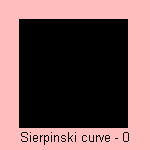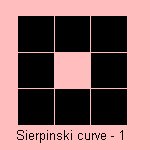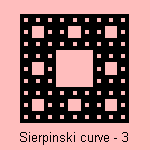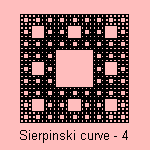The curve is also known as the Sierpinski (universal plane) curve, Sierpinski square or the Sierpinski carpet.
It has named after the Polish mathematician Waclaw Sierpinski (1882-1969), but it was Stefan Mazurkiewicz who found the curve (in 1913).

The curve is the only plane locally connected one-dimensional continuum S such that the boundary of each complementary domain of S is a simple closed curve and no two of these complementary domain boundaries intersect.
Wow!
In other words: the Sierpinski curve contains a topologically equivalent copy of any compact one-dimensional object in the plane.

The fractal dimension of the curve is equal to log 8/ log 3, i.e. about 1.8928 1).
The curve is a two-dimensional generalization of the Cantor set.

Some kind of shells (conus textilus, conus gloriatnatis) have patterns that resemble the Sierpinksi square.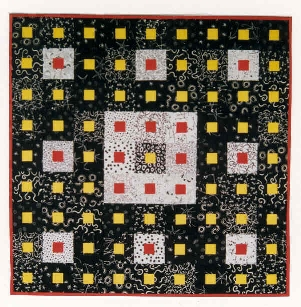Professor Gerda de Vries of the University of Alberta designed a quilt named ´Sierpinksi Meets Mondrian´, based on the Sierpinski curve. The quilt was made in 2002 in response to the Edmonton & District Quilters' Guild challenge to create a quilt in the theme ´Voices in Cloth´. It was made for the entry category ´A Picture is Worth a Thousand Words´.

notes

1) Fractal dimension = log N / log e, where N is the number of line segments and e the magnification.
For the Sierpinski curve: N=8, e=3.